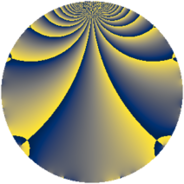# Properties

 Label 3549.2.lLevel $3549$ Weight $2$ Character orbit 3549.l Rep. character $\chi_{3549}(529,\cdot)$ Character field $\Q(\zeta_{3})$ Dimension $410$ Sturm bound $970$

# Related objects

## Defining parameters

 Level: $$N$$ $$=$$ $$3549 = 3 \cdot 7 \cdot 13^{2}$$ Weight: $$k$$ $$=$$ $$2$$ Character orbit: $$[\chi]$$ $$=$$ 3549.l (of order $$3$$ and degree $$2$$) Character conductor: $$\operatorname{cond}(\chi)$$ $$=$$ $$91$$ Character field: $$\Q(\zeta_{3})$$ Sturm bound: $$970$$

## Dimensions

The following table gives the dimensions of various subspaces of $$M_{2}(3549, [\chi])$$.

Total New Old
Modular forms 1026 410 616
Cusp forms 914 410 504
Eisenstein series 112 0 112

## Trace form

 $$410q + 3q^{3} + 408q^{4} - 205q^{9} + O(q^{10})$$ $$410q + 3q^{3} + 408q^{4} - 205q^{9} + 8q^{10} + 4q^{11} + 8q^{12} + 20q^{14} + 388q^{16} + 16q^{17} + 3q^{19} + 8q^{20} + 5q^{21} - 2q^{22} - 16q^{23} - 12q^{24} - 195q^{25} - 6q^{27} + 16q^{28} + 11q^{31} + 40q^{32} + 56q^{34} - 10q^{35} - 204q^{36} + 46q^{37} - 28q^{38} + 34q^{40} - 18q^{41} + 14q^{42} + 20q^{44} - 16q^{46} - 12q^{47} + 18q^{48} + 2q^{49} + 2q^{50} - 12q^{51} - 32q^{53} + 30q^{55} + 86q^{56} + 38q^{57} - 20q^{58} - 64q^{59} - 32q^{60} + 5q^{61} - 8q^{62} - 3q^{63} + 412q^{64} + 16q^{66} - 17q^{67} + 88q^{68} + 8q^{69} - 76q^{70} - 10q^{71} - 4q^{73} + 24q^{74} - 58q^{75} + 12q^{76} + 26q^{77} + 19q^{79} - 20q^{80} - 205q^{81} + 14q^{82} - 64q^{83} + 8q^{84} + 20q^{85} - 20q^{86} - 60q^{87} - 24q^{88} + 32q^{89} - 16q^{90} - 28q^{92} - 34q^{93} + 60q^{94} - 112q^{95} - 14q^{96} - 23q^{97} + 58q^{98} - 8q^{99} + O(q^{100})$$

## Decomposition of $$S_{2}^{\mathrm{new}}(3549, [\chi])$$ into newform subspaces

The newforms in this space have not yet been added to the LMFDB.

## Decomposition of $$S_{2}^{\mathrm{old}}(3549, [\chi])$$ into lower level spaces

$$S_{2}^{\mathrm{old}}(3549, [\chi]) \cong$$ $$S_{2}^{\mathrm{new}}(91, [\chi])$$$$^{\oplus 4}$$$$\oplus$$$$S_{2}^{\mathrm{new}}(273, [\chi])$$$$^{\oplus 2}$$$$\oplus$$$$S_{2}^{\mathrm{new}}(1183, [\chi])$$$$^{\oplus 2}$$